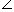# Formulas To Solve Height And Distance Questions

## Height and Distance Formulas

There are basically two terms associated with heights and distances which are as follows (i) Angle of Elevation.
(ii) Angle of Depression.

Read Also – Tips and Tricks of Heights and Distance questions.

### Formulas for Angle of Elevation

Suppose a man is watching an object which is at some height say the top of the building. The line joining the eye of the man with the top of the building is called the line of sight. The angle made by the line of sight with the horizontal line is called angle of elevation.
Angle of elevation of P from O =AOP.### Formulas for Angle of Depression

Suppose a man looks down at an object while standing at some height. The line joining the eye of the man with the bottom of the object is called the line of sight. The angle made by the line of sight with the horizontal line is called angle of depression.## Height and Distance Formulas for Trigonometric ratio

1.  ΔABC is a right angled triangle where is AB is the perpendicular, AC is the hypotenuse, and BC is the base.

Then,

• Sin θ  = AB/AC
• Cos θ = BC/AC
• Tan θ  = AB/BC
• Cosec θ  = AC/AB
• Sec θ  = AC/BC
• Cot θ  = BC/AB

Trigonometrical Identities:

• sin2θ + cos2 θ = 1
• 1 + tan2θ = sec2 θ
• 1 + cot2θ = cosec2 θ